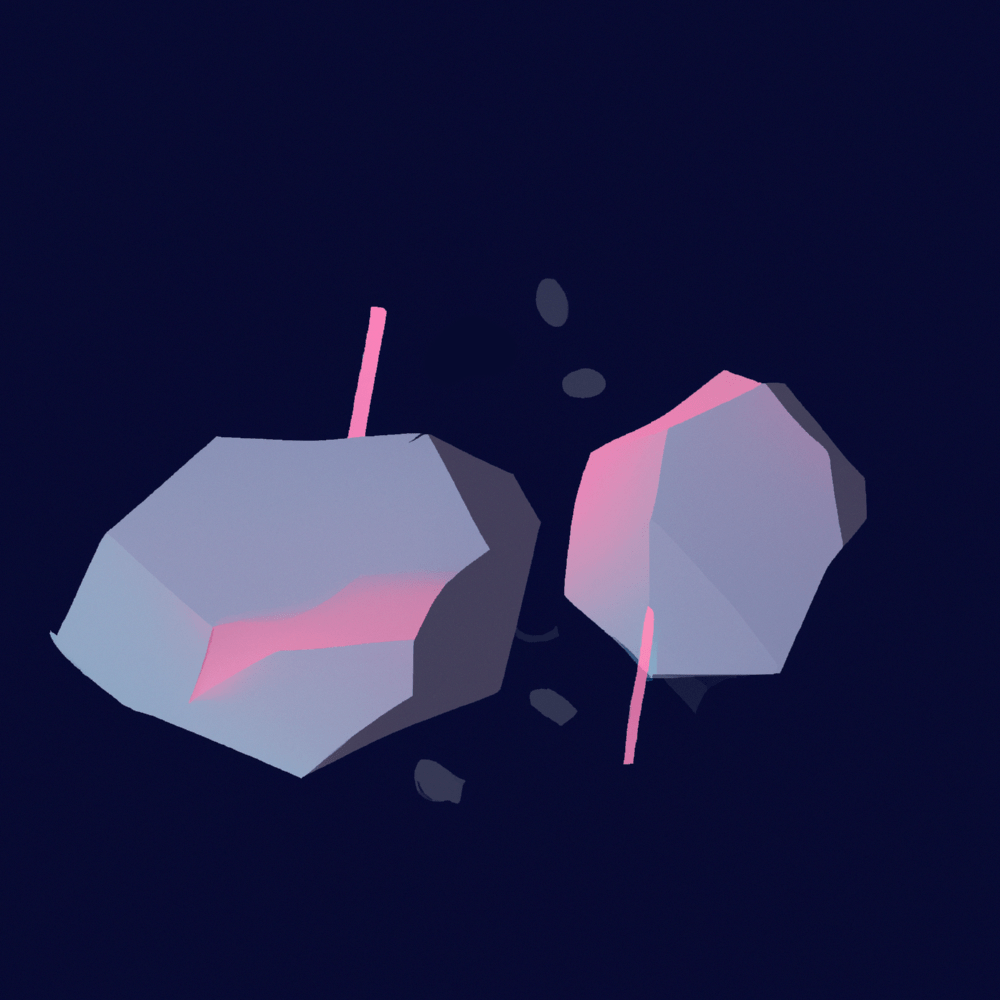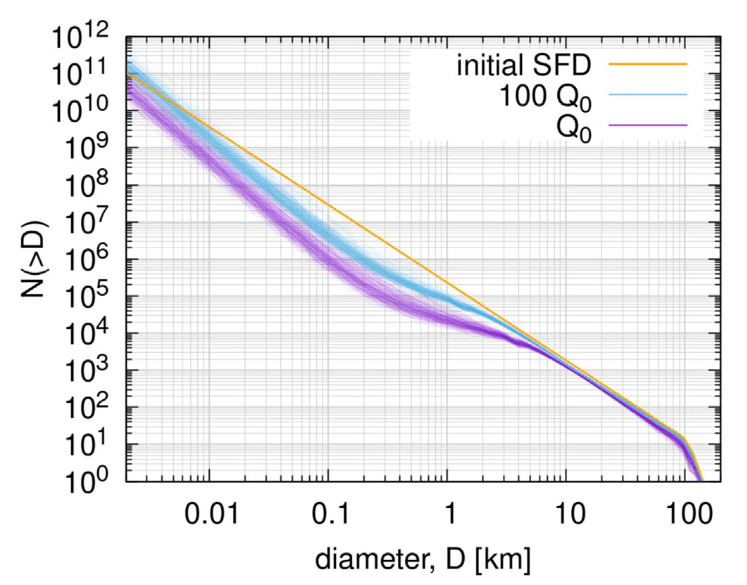# Collisional Evolution← back to the research overview

see publications →

### 1. Introduction

Collisions between small (sub-micron) dust and ice grains in the early proto-planetary disk allow the grains to grow to centimetre-scaled particles – often referred to as ‘pebbles’ (e.g., Dominik and Tielens, 1997; Blum and Wurm, 2000; Wada et al., 2009; Güttler et al., 2010). When sufficiently concentrated within the disk, pebbles form a pebble cloud that can gently collapse under its own gravity. The current preferred and most-studied mechanism that can lead to such a pebble concentration is the streaming instability (e.g., Youdin and Goodman, 2005; Johansen et al., 2007; Wahlberg Jansson and Johansen, 2014; Simon et al., 2017) which is expected to mostly form large (∼100 km) planetesimals (e.g. Simon et al., 2016; Schäfer et al., 2017; Klahr and Schreiber, 2020).

Yet, although small bodies form big most of them observed today — especially comets — have typical diameters between 1 and 10 km. Thus, the original planetesimals get disrupted during collisions in a final formation step. We can study this process by using collisional evolution codes like Boulder (Morbidelli et al., 2009; Nesvorný et al., 2011). Understanding this process in crucial in reconstructing the history of these relics of the early Solar System and thus informing us on the era of planet formation.

### 2. Strength of small bodies

A crucial property to understand the collisional evolution of small bodies is their strength. The strength of a body in this context is quantified by$Q^*_D$ (see Fig. 1 below).$Q^*_D$ is the energy per mass needed to disrupt and disperse 50% of a target body. The negative slope at small and positive slope at large sizes indicate that bodies get stronger when they get larger/smaller, but there is a weakest body in the middle. The point where$Q^*_D$ reaches a minimum is the diameter of a body that is easiest to disrupt. For asteroids, this is around 200 m, whilst for trans-Neptunian objects, we now believe the weakest body to be around 20 m (Bottke et al. 2023).Figure 1. Critical impact energy, , according to different studies (BA99; Benz & Asphaug 1999; LS09 Leinhardt & Stewart 2009; B05 Bottke et al. 2005; B20 Bottke et al. 2020; J10 Jutzi et al. 2010) are shown in the left panel. The right panel shows three different model assumptions (dashed green), (dotted green), and (solid green) as well as a with a minimum of 20 m (purple line). In addition, the literature values from Benz & Asphaug (1999), blue, and Leinhardt & Stewart (2009), orange, are shown as a reference. Figure taken from Marschall et al. 2022.

### 3. The collisional evolution of the size distribution

I have used the Boulder collisional code to study the collisional evolution of Jupiter Trojans (Marschall et al. 2022) and Kuiper Belt Objects (Bottke et al. 2023). Figure 2 below shows an example of how the initial Jupiter Trojan size frequency distribution (orange) changes over 4.5 billion years depending on the strength of the material (blue and purple). As can be expected, overall, weaker material$Q^*_D = Q_0$ erodes the population more than stronger material$Q^*_D = 100 Q_0$. Furthermore, at smaller sizes (diameters smaller than about 2 km), the stochastic nature of collisions plays an important role. The different lines illustrate this, each representing a different random path of collisions. This results in large — roughly one order of magnitude — uncertainty in the number of objects at small sizes, e.g., 100 meters.Figure 2. The initial Trojan SFD is shown in orange. The faint lines in purple are 300 different SFDs after 4.5 Gy of collisional grinding, assuming , while the blue lines assume . Figure taken from Marschall et al. 2022.

see publications →